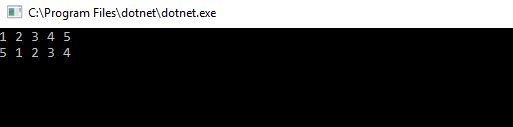Posted on

## Array Left Rotation by D – C# Code

left rotation operation on an array of size n shifts each of the array’s elements 1 unit to the left. For example, if 2 left rotations are performed on array [1,2,3,4,5], then the array would become  [3,4,5,1,2].

Given an array of  n integers and a number, d , perform d left rotations on the array. Then print the updated array as a single line of space-separated integers.

Input Format

The first line contains two space-separated integers denoting the respective values of  (the number of integers) and  (the number of left rotations you must perform).
The second line contains  space-separated integers describing the respective elements of the array’s initial state.

Output Format

Print a single line of  space-separated integers denoting the final state of the array after performing  left rotations.

Sample Input

5 4
1 2 3 4 5


Sample Output

5 1 2 3 4

ExplanationCode

 static int[] LeftRotationByD(int[] a,int k)
{
int[] b = new int[a.Length];

int index;
int length = a.Length;
int place;

for (int i = 0; i < length; i++)
{
index = i - k;
place = length + index;
if (index >= 0)
{
b[index] = a[i];
}
else
{
b[place] = a[i];
}
}
return b;

}


We can see the results for 1 and 4 rotations as follows: